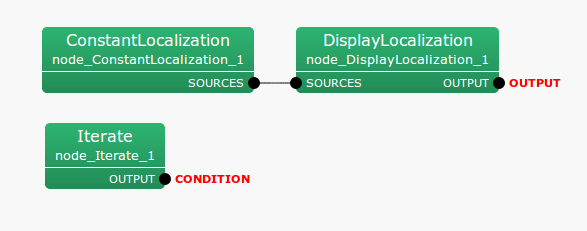## 6.2.12 ConstantLocalization

### 6.2.12.1 Outline of the node

A node that continuously outputs constant sound source localization results. There are four parameters used for this node, which are ANGLES, ELEVATIONS, POWER, and MIN_ID, and the user sets azimuths (ANGLES), elevation angles (ELEVATIONS), power (POWER), and IDs (MIN_ID) of the sound sources. Since each of these parameters is Vector , multiple localization results can be output.

### 6.2.12.2 Necessary file

No files are required.

### 6.2.12.3 Usage

When to use

This node is used in the case that the user wishes to perform evaluations when a source localization result is already known. For example, when wishing to judge whether a problem is in the separation processing or are sound source localization errors, or wishing to evaluate the performance of sound separation under the same sound source localization condition, while evaluating the results of sound source separation.

Typical connection

Figure 6.26 and 6.27 show a connection example. This network continuously displays constant localization results.Figure 6.26: Connection example of ConstantLocalization  1Figure 6.27: Connection example of ConstantLocalization  2

### 6.2.12.4 Input-output and property of the node

Input

No inputs.

Output

SOURCES

: Vector< ObjectRef >  type. Fixed sound source localization results are output. The data ObjectRef  refers to Source  type data.

Parameter

Table 6.26: Parameter list of ConstantLocalization
 Parameter name Type Default value Unit Description ANGLES > [deg] Azimuth (right or left) of the sound source ELEVATIONS > [deg] Elevation angle (up or down) of the sound source POWER > Power of the sound sources MIN_ID 0 IDs of the sound sources
ANGLES

: Vector< float >  type. Azimuth (right or left) of the direction that the sound source comes in. The unit of angle is degree.

ELEVATIONS

: Vector< float >  type. Elevation angle (up or down) of the direction that the sound source comes from. The unit of angle is degree.

POWER

: Vector< float >  type. This sets the power of sound sources. The unit is dB, same as the spatial spectrum calculated in LocalizeMUSIC . This parameter is not mandatory. If nothing defined, POWER is automatically set as 1.0.

MIN_ID

: int type. This sets the minimum ID number allocated to each sound source. The IDs should be unique in order to distinguish each sound source. The IDs are allocated in order of elements specified in ANGLES and ELEVATIONS, whose number if started from MIN_ID. For instance, if MIN_ID = 0 and ANGLES = <Vector<float> 0 30> are specified, the ID of $0^\circ$ sound source will be zero, and the ID of $30^\circ$ sound source will be one.

### 6.2.12.5 Details of the node

It is assumed that the number of sound sources is $N$, the azimuth (ANGLE) of the $i$ th sound source is $a_ i$ and the elevation angle (ELEVATION) is $e_ i$. Here, parameters are described as follows.

ANGLES:

< Vector< float >  $a_1$ $\dots$ $a_ N$>

ELEVATIONS:

< Vector< float >  $e_1$ $\dots$ $e_ N$>

In this way, inputs are performed based on a spherical coordinate system, though the data that ConstantLocalization  actually outputs are values in the Cartesian coordinate system $(x_ i, y_ i, z_ i)$, which correspond to points on the unit ball. Conversion from the spherical coordinate system to the Cartesian coordinate system is performed based on the following equations.

 $\displaystyle x_ i$ $\displaystyle =$ $\displaystyle \cos ( a_ i \pi / 180 ) \cos ( e_ i \pi / 180 )$ (5) $\displaystyle y_ i$ $\displaystyle =$ $\displaystyle \sin ( a_ i \pi / 180 ) \cos ( e_ i \pi / 180 )$ (6) $\displaystyle z_ i$ $\displaystyle =$ $\displaystyle \sin ( e_ i \pi / 180 )$ (7)

Other than the coordinates of sound sources, ConstantLocalization  also outputs the power (specified by POWER. If not set, fixed at $1.0$) and ID (specified by MIN_ID + $i$) of the sound source.